#### You may also like### Exploring Wild & Wonderful Number Patterns

EWWNP means Exploring Wild and Wonderful Number Patterns Created by Yourself! Investigate what happens if we create number patterns using some simple rules.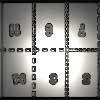### Magazines

Let's suppose that you are going to have a magazine which has 16 pages of A5 size. Can you find some different ways to make these pages? Investigate the pattern for each if you number the pages.### Pebbles

Place four pebbles on the sand in the form of a square. Keep adding as few pebbles as necessary to double the area. How many extra pebbles are added each time?

# Swimming Pool Tiles

##### Age 7 to 11Challenge Level
Mr Garrod from Waverley Primary School wrote to share how his class had worked on this task:

We really enjoyed looking at this problem. The children observed a variety of patterns emerging from different number series. It was especially useful to highlight the difference between values. Here are their findings, as we recorded them as a class group:

- The number of tiles between square numbers increases by a difference of two each time (e.g. the tile difference between 1 and 4 is two blank tiles, between 4 and 9 is 4, between 9 and 16 is 6 tiles, and so on).
- Odd and even numbers create an alternating checked pattern.
- The diagonals in from 200 are all even; the diagonals in from 199 are all odd.
- Multiples of 4 create perfect patterns of diagonals across the tiled area.
- With prime numbers, there is an alternating difference of 1 and 3 tiles between each entry in the series (e.g. between 3 and 5 is one tile, between 5 and 7 is one tile, between 7 and 11 is three tiles, between 11 and 13 is one, 13 and 17 is three, 17 and 19 is one, etc).
- In the diagonal rows, there is an alternating pattern of units (e.g. from 191 to 153 diagonally: 1713317133)
- Triangular numbers give a steady incremental tile difference between each entry (1 tile difference, 2 tile difference, 3 tile difference, etc).

There is probably a lot more to find, but these became apparent after our first lesson. We put a series of the 100 squares provided onto large sheets of sugar paper and worked from this is groups.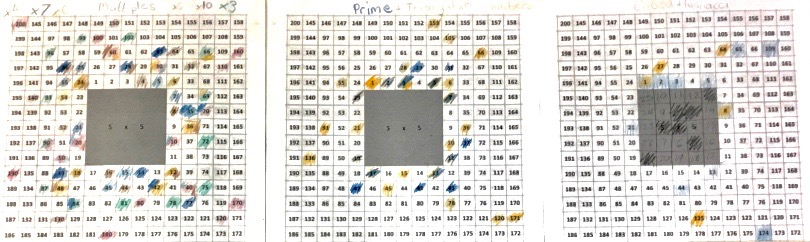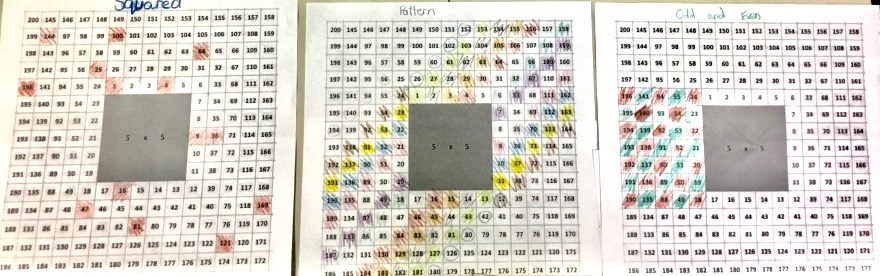The above images may be viewed more clearly here and here

Four pupils from Rowan Class at Stourport Primary Academy submitted their work:

Daisy
The following photos show some of the patterns I discovered when I tried the Swimming Pool Tiles problem. I couldn't see an obvious pattern to the 5 times table, but once I had highlighted all the numbers in the 3 times table I noticed that you could join these up in groups of three to make a pattern of rotating triangles.
After I had completed this I wondered why there was this obvious pattern of triangles with the 3 x table but no obvious pattern with the 5 x table. I wonder whether there would be a clearer pattern if I tried the 5 x table on a bigger grid.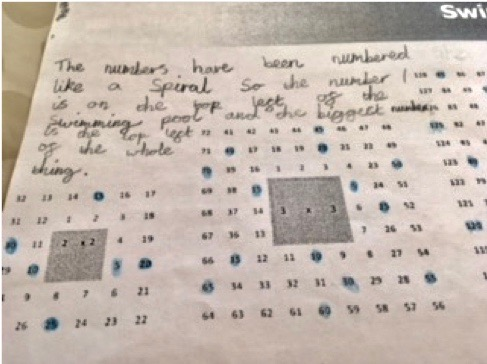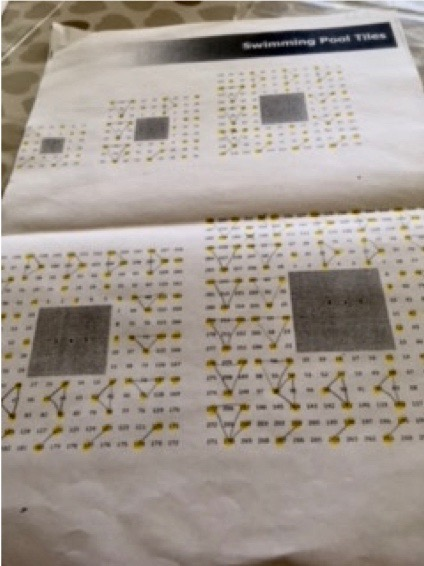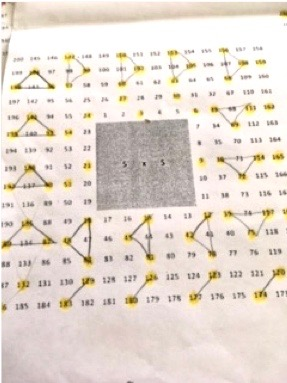Lois
I decided to colour all the multiples of 9 on the different sized grids. I noticed that if you join all the numbers with lines (so you would join 9 to 18, then 18 to 27 and so on), there is a pattern where it makes a spiral of shapes. It goes from a triangle to a square to a pentagon and so on so the shapes within the spiral increase by one corner each time. Here are the photos of my work to prove it! Originally I had made a prediction that odd-numbered times tables wouldn't have a pattern. I was wrong!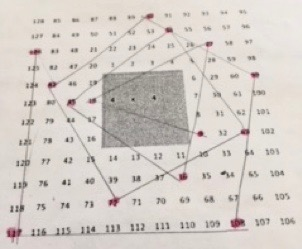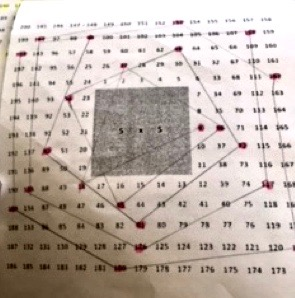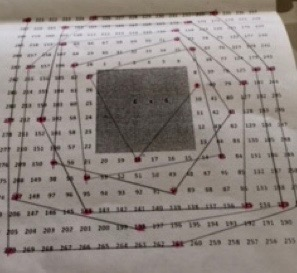Keira
Most of my friends said that there isn't a pattern for the 5 x-table but I thought you could group the highlighted numbers together and make petals on a flower like this. My group had a go, with help from our teacher, at working out how you could predict how many tiles would be needed to go around different pool sizes. One of my friends spotted that if you multiply the area of the pool by 8 you get the number of tiles needed to go around it. For example, if the pool is 3 x 3 you would do 3x3=9 then multiply 9 by 8 which gives you 72 tiles.  We checked this with all the tiles on the sheet and found this to be true. Then we used the same formula to calculate how many tiles would surround a pool that is 100 x 100.
100 x 100 = 10000.    10000 x 8 = 80000. We decided not to have a go at drawing that one!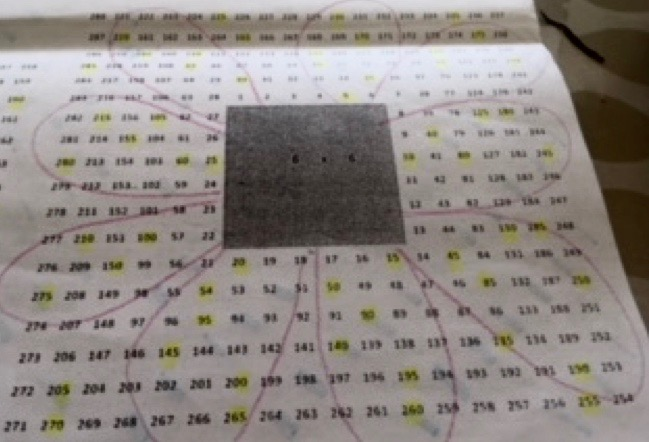Tom describes what he did:
I decided to investigate the 4 times table and discovered a diagonal pattern.
Next, I decided to colour the 4s, 8s and 12s on the same grid.  Exactly the same numbers were coloured because of course the 8 and 12 times tables are also multiples of 4. I also spotted that if you join up the diagonal lines and join up the sides you make six isosceles trapeziums. This lesson was one of my favourites ever maths lessons.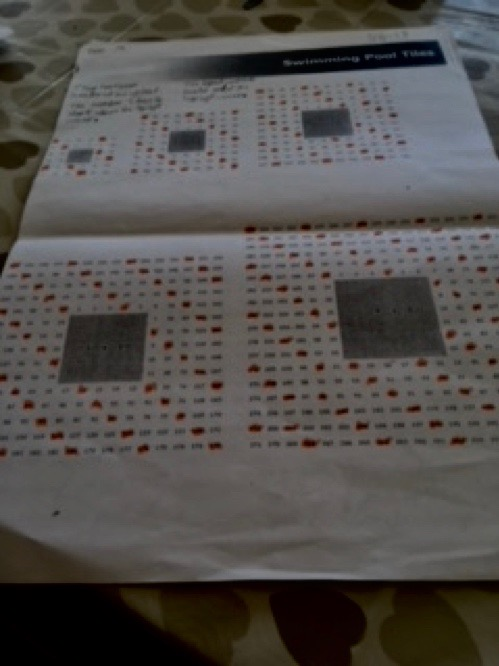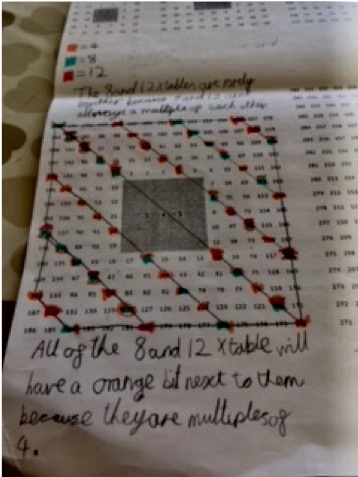These pieces of work are fantastic and suggest you were curious to find out more, as well as being resilient as you had a mathematical adventure. The work of the Stourport pupils and particularly their images can be more easily viewed here: All Stourport Swimming Pool Tiles.doc